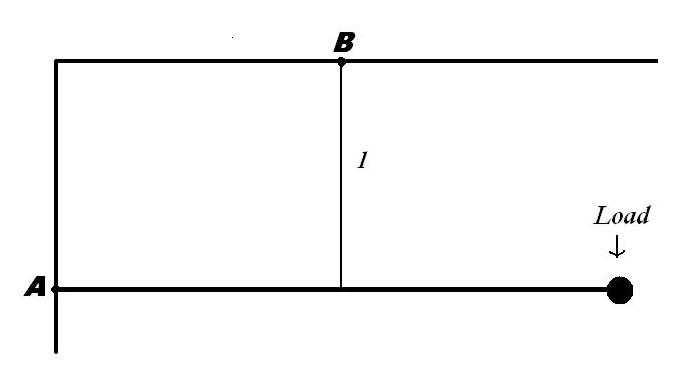# Is it S.H.M?

A weightless rigid rod with a load at the end is hinged at point $A$ to the walls so that it can rotate in all directions. The rod is kept in the horizontal position by a vertical in-extensible thread of length $l$, fixed at its midpoint. The load receives a momentum in the direction perpendicular to the plane of the figure (which is shown below).If the period $T$ of small oscillations of the system can be described as
$2\pi$$\dfrac{\sqrt{kl}}{\sqrt{g}}$ Find the value of $k$

×

Problem Loading...

Note Loading...

Set Loading...